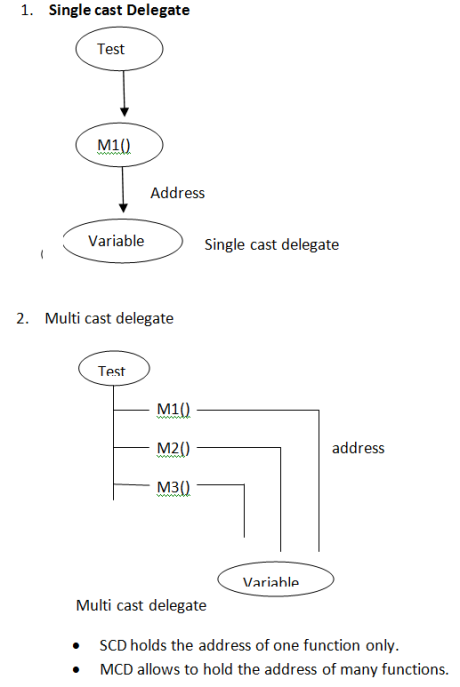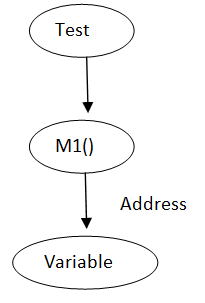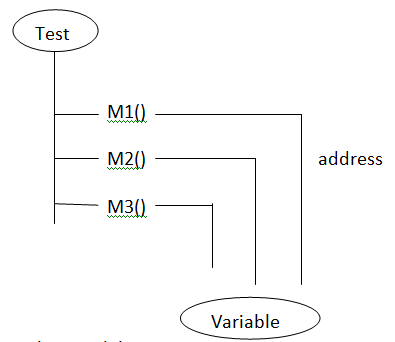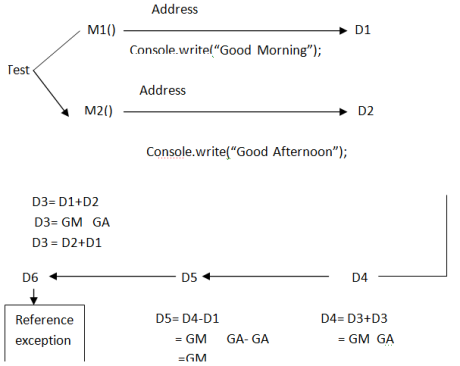# Working with Delegates in C# .net

Blog Author

Tekslate

Published Date

16th October, 2020

Ratings

Views

291

## Working with Delegates in C# .net1. Delegates hold the address of one function or address of many functions.

### Delegates are divided into two types:-

1. Single cast Delegate### Multicast delegate• SCD holds the address of one function only.
• MCD allows holding the address of many functions.

### 4.Steps to crates a Delegate:-

Step 1.  Write a class with a method

Syntax:-

Class Test {

Public void Print ()

{

logic ;

}

}

Step 2. Create a Delegate

Syntax :- public delegate void D name ();

• Keyword
• Delegate name

Step 3 . create an object for the delegate with the address of a method.

Syntax :-

Test t  = new Test ()

D name  d = new D name  (t. print);

Step 4. Call the Delegate

Syntax :-  d ();

Example on SCD :-

• Open console Application Project
• Code in GD (before Main ())
``````{

Class Test

{

Public void Print ()

{

Console .Write (“From Print”);

}

}

//test

Public delegate void D name ();

Code in main ()

Test t  = new Test ();

D name d = new D name (t. print);

D();

``````

OBS:-

Delegates encapsulate some information like class name and method names.

### Working with Multicast Delegates:-

1. MCD holds the addresses of many functions.
2. MCD holds the sequence of functions
3. MCD supports arithmetic + and _ operations.
4. ‘+’ operator add a function in to the sequence.
5. ‘_’ operator removes a function from the sequence.

OBS:D6= D5 – D3 – D2 = GM  GA  - GM  GA  - GA = GM

### Example on MCD :-

• open console Application Project
• Code for GD (before Main ())
`````` Class Test

{

Public void M1 ()

{

Console . Write (“GM     ”);

}

//M1

Public void M2 ()

{

Console . Write Line (“GA”);

}

//M2

}

// Test

Public delegate void XYZ ();

Static void Main  (Strings []  args)

``````
``````Code in main ()

{

Test t = new Test ();

XYZ  = d1, d2, d3,d4,d5,d6;

D1 = new XYZ (t. M1);

D2 = new XYZ (t.M2);

D3 = d1 + d2; Console. Write line (“From D3 :”);

D3 ();

D4 = d3 +d3;

Console. Write line (“From D4 :”);

D4 ();

D5 = d4- d1;

Console. Write line (“From D5:”); D5 ();

D6 =d5-d3-d2;

Console. Write line (“From D6 :”);

D6 ();

}  ``````